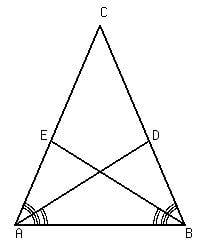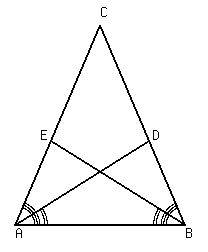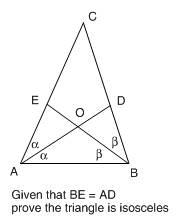# All Triangles are Isosceles Proof (Euclidean Geometry)

Menaus
Prove that in any triangle, if the angle bisectors of two angles are congruent, then the triangle is isosceles

Before I give my proof, here is a lemma to it:

If a pair of vertical angles both have angle bisectors, then all resulting angles are congruent.Given: Vertical Angles $∠2$ and $∠4,$ and $∠1$ and $∠3,$ Angle bisectors such that $∠1 \cong ∠2$, and $∠3 \cong ∠4$

By vertical angle theorem, ∠1 and ∠3 must be congruent, as well as ∠2 and ∠4.

∠3 and ∠4, must be congruent, ∠1 and ∠2 must also be congruent by the definition of angle bisector.

By the transitive property of congruent angles theorem, ∠1 and ∠4 must be congruent, and ∠2 and ∠3 must also be congruent.

Therefor, all angles must be congruent to each other.

This concludes the lemma, here is my proof. Please poof read it... No pun intended. ;)

In any triangle, if the angle bisectors of two angles are congruent, then the triangle is isoscelesGiven: $ΔABC, ∠EAD\cong∠EAB, ∠DBE\cong∠DBA,$ and $AD = BE$

Let there exist a line through FO (where O is the point in which AD, and BE intersect) such that line FO is congruent to segment AB

Let there exist a point G where line GB is perpendicular to segment AB.

(Please note, for the purposes of this proof, there need not be a line perpendicular to segment AB, I am simply trying to show where G is, in relation to all other parts of the diagram)

∠AOF, ∠FOE, ∠BOG, and ∠GOD are all congruent to each other by my lemma, I'll call it the Vertical ∠ Bisector Theorem.

∠OBA is congruent to ∠FOE by PAI theorem.

∠GOD is congruent to ∠AOF by PAI theorem

By the transitive law of congruent angles, ∠OBA and ∠OAB are congruent

By the transitive law of congruent angles, ∠CAB, and ∠CBA are congruent

Congruent angles imply congruent segments, therefor, AC = BC

quod erat demonstrandum

Is my proof correct? Do I need to be more rigorous? What problems are there?

Last edited:

dirk_mec1
Use standard format of PF!

Homework Helper
Gold Member
I didn't read your whole proof since it seems pointless. If the bisector angles are equal then the angles themselves are equal and the triangle is isosceles. Or maybe I misunderstand what you are claiming.

Menaus
Yes, while that is true, I simply proved it in a different way.

I'll remind you this is a point in the math book where it asks me to prove "if the angle bisectors are equal, prove the triangle is isosceles" (So I have to prove it, I can't just say 'its obvious!' ;) ). I simply saw a different rout, and took it. I'd just like to know if I am correct.

Homework Helper
Gold Member
Yes, while that is true, I simply proved it in a different way.

I'll remind you this is a point in the math book where it asks me to prove "if the angle bisectors are equal, prove the triangle is isosceles" (So I have to prove it, I can't just say 'its obvious!' ;) ). I simply saw a different rout, and took it. I'd just like to know if I am correct.

I just tried to quote your original post, which doesn't work. So I share the sentiments of the post #2.

Just a couple more comments. In your hypotheses you have angle EAD = angle EAB which is clearly false, and so is the next one.

Also what exactly does it mean when you say "the angle bisectors are equal".

Is what you are trying to prove that if two angles of a triangle are equal, then the triangle is isosceles?

Menaus
I just tried to quote your original post, which doesn't work. So I share the sentiments of the post #2.

Just a couple more comments. In your hypotheses you have angle EAD = angle EAB which is clearly false, and so is the next one.

This is what is given in the textbook question. I find it easy to believe it is true because of the definition of angle bisector.

"A Bisector is the division of something into two equal or congruent parts, usually by a line, which is then called a bisector"

In this case it is an angle bisector, therefor, it divides the angles into two equal parts. It is impossible that EAD != EAB

Also what exactly does it mean when you say "the angle bisectors are equal".

Is what you are trying to prove that if two angles of a triangle are equal, then the triangle is isosceles?

Lets say that points A and D, and points B and E all have numerical values a, d, b, and e, respectively. If AD = BE, then

|d - a| = |e - b|

I.E. their lengths are equal.

And I am trying to prove that "In any triangle, if the angle bisectors of two angles are congruent, then the triangle is isosceles"

That means I'm proving that if AD = BE, and EAD = EAB, as well as DBE = DBA, then AC = BC

Menaus
One more thing, after reading over my proof, I made a mistake:

"line FO is congruent to segment AB"

I meant to say that line FO is parallel to segment AB

Homework Helper
Gold Member
Just a couple more comments. In your hypotheses you have angle EAD = angle EAB which is clearly false, and so is the next one.

This is what is given in the textbook question. I find it easy to believe it is true because of the definition of angle bisector.

"A Bisector is the division of something into two equal or congruent parts, usually by a line, which is then called a bisector"

In this case it is an angle bisector, therefor, it divides the angles into two equal parts. It is impossible that EAD != EAB

I guess I am wasting my time here. In your picture it is obvious that EAD != EAB. I'm gone from this thread as of now.

Menaus
In geometry we don't give 'its obvious' as a proof. I'm fine with accepting that this is not true in my picture, just prove it, and I can use a different picture...

Homework Helper
Gold Member
In geometry we don't give 'its obvious' as a proof. I'm fine with accepting that this is not true in my picture, just prove it, and I can use a different picture...

But it is your hypothesis that EAD=EAB so how can it be fine with you that they aren't equal. Your picture needs to satisfy your hypothesis. So draw a different picture if you can.

I think you are just trolling and I really am leaving this thread for good. Maybe someone else will be interested.

Menaus
But it is your hypothesis that EAD=EAB so how can it be fine with you that they aren't equal. Your picture needs to satisfy your hypothesis. So draw a different picture if you can.

"I'm fine with accepting that this is not true in my picture"

Really you just restated what I just said.

I think you are just trolling and I really am leaving this thread for good. Maybe someone else will be interested.

I can be fine with EAD != EAB in my picture. My picture is just there to help people better visualize things, the picture itself says nothing about the proof.

Again, I'd like for you to prove it, otherwise it is irrelevant in geometry. Or any theory, really.

I'm not trolling, as I would really like to see what problems may be in my proof, but up till now the points you have made against it are invalid.

Staff Emeritus
Homework Helper
test
Prove that in any triangle, if the angle bisectors of two angles are congruent, then the triangle is isosceles

Before I give my proof, here is a lemma to it:

If a pair of vertical angles both have angle bisectors, then all resulting angles are congruent.
Okay. I had to think a while to realize what you meant by "all resulting angles". You really mean the 4 angles formed by the bisection of the two vertical angles. A figure would have been helpful here.Given: Vertical Angles $∠2$ and $∠4,$ and $∠1$ and $∠3,$ Angle bisectors such that $∠1 \cong ∠2$, and $∠3 \cong ∠4$

By vertical angle theorem, ∠1 and ∠3 must be congruent, as well as ∠2 and ∠4.

∠3 and ∠4, must be congruent, ∠1 and ∠2 must also be congruent by the definition of angle bisector.

By the transitive property of congruent angles theorem, ∠1 and ∠4 must be congruent, and ∠2 and ∠3 must also be congruent.

Therefor, all angles must be congruent to each other.

This concludes the lemma, here is my proof. Please poof read it... No pun intended. ;)

In any triangle, if the angle bisectors of two angles are congruent, then the triangle is isoscelesGiven: $ΔABC, ∠EAD\cong∠EAB, ∠DBE\cong∠DBA,$ and $AD = BE$
As was pointed out already -- these angles, as shown in your figure, are not congruent. Surely you can see this? You really can't excuse it by saying "the picture itself says nothing about the proof". The picture defines where points E and D are, and you use those points when referring to the angles involved.

Once drawn and used to define points like D and E, your proof can't then contain statements that clearly contradict the figure. The proof and the figure go hand in hand.

Let there exist a line through FO (where O is the point in which AD, and BE intersect) such that line FO is [STRIKE]congruent[/STRIKE] parallel to segment AB
Okay. I have added your correction about line FO here. It would have been a good idea to show points O and F in the figure.
Let there exist a point G where line GB is perpendicular to segment AB.

(Please note, for the purposes of this proof, there need not be a line perpendicular to segment AB, I am simply trying to show where G is, in relation to all other parts of the diagram)
In the next step, one must assume that ray OG bisects ∠BOD in order to use your lemma. That should have been specified here. Again, showing point G (along with O and F) in the figure would be a big help to people trying to follow your proof and help you out.

So, at this point my mental picture has O where you said it was. FG form a segement with O between F and G; F is to the left of O, G is to the right, and line FG (or FO or OG) is parallel to AB.
∠AOF, ∠FOE, ∠BOG, and ∠GOD are all congruent to each other by my lemma, I'll call it the Vertical ∠ Bisector Theorem.

∠OBA is congruent to ∠FOE by PAI theorem.
They are congruent, but not by that theorem, which relates to alternate interior angles. They are congruent because they are corresponding angles -- if my mental picture description is correct.

∠GOD is congruent to ∠AOF by PAI theorem
No, they are congruent by your lemma, as you have already stated.
By the transitive law of congruent angles, ∠OBA and ∠OAB are congruent
[Edit: the following is incorrect. My apologies.] [STRIKE]It was unnecessary to go through all those steps to prove this statement. This statement is simply a given. From your figure, ∠OBA and ∠OAB are the assumed congruent bisectors of angles ∠CAB and ∠CBA, as needed in order to construct the proof.[/STRIKE]
By the transitive law of congruent angles, ∠CAB, and ∠CBA are congruent
No, it doesn't follow form the transitive law. To use that law, you need to have shown that ∠CAB and ∠CBA are congruent to some other angle, such as ∠AOE. You have not done that.
Congruent angles imply congruent segments, therefor, AC = BC[/b]

quod erat demonstrandum

Is my proof correct? Do I need to be more rigorous? What problems are there?

Last edited:
Menaus
Thank you for coming back and looking at my proof. Sorry if I was acting in a way that looked 'trollish'

As was pointed out already -- these angles, as shown in your figure, are not congruent. Surely you can see this? You really can't excuse it by saying "the picture itself says nothing about the proof". The picture defines where points E and D are, and you use those points when referring to the angles involved.

Once drawn and used to define points like D and E, your proof can't then contain statements that clearly contradict the figure. The proof and the figure go hand in hand.

I suppose I'll have to draw it myself, then. I was hoping I could find a suitable figure online, but I guess not.

Okay. I have added your correction about line FO here. It would have been a good idea to show points O and F in the figure.

...

In the next step, one must assume that ray OG bisects ∠BOD in order to use your lemma. That should have been specified here. Again, showing point G (along with O and F) in the figure would be a big help to people trying to follow your proof and help you out.

So, at this point my mental picture has O where you said it was. FG form a segement with O between F and G; F is to the left of O, G is to the right, and line FG (or FO or OG) is parallel to AB.

Like I said earlier, I found the figure online, and it approximated the original figure in the textbook so well, that I decided to use it. I'll correct this and create my own figure in MS paint or something. ;)

Anyway, give me some time and I'll post back with my updated proof. Thank you again.

Homework Helper
Gold Member
Since you have finally acknowledged the problem with the angles I will give you a correct picture:The graphic states what I believe you are trying to prove. Fair warning: That is a known difficult theorem to which you are unlikely to find a simple purely geometric solution.

Staff Emeritus
Homework HelperThe graphic states what I believe you are trying to prove. Fair warning: That is a known difficult theorem to which you are unlikely to find a simple purely geometric solution.
Wait, I thought we are given that $\alpha = \beta$, not BE=AD? [Edit: LCKurtz is correct, I am mistaken here]

Menaus said:
... if the angle bisectors of two angles are congruent, then the triangle is isosceles
[STRIKE]
This may seem really basic to those of us who are well into our careers, but it is a reasonable exercise for somebody just learning to do proofs for the first time.[/STRIKE] [Edit: another incorrect statement]

Last edited:
Homework Helper
Gold Member
Prove that in any triangle, if the angle bisectors of two angles are congruent, then the triangle is isosceles

This concludes the lemma, here is my proof. Please poof read it... No pun intended. ;)

In any triangle, if the angle bisectors of two angles are congruent, then the triangle is isoscelesGiven: $ΔABC, ∠EAD\cong∠EAB, ∠DBE\cong∠DBA,$ and $\color{red}{AD = BE}$

Wait, I thought we are given that $\alpha = \beta$, not BE=AD?

This may seem really basic to those of us who are well into our careers, but it is a reasonable exercise for somebody just learning to do proofs for the first time.

Surely part of the problem here is you can't figure out what he is assuming or purporting to prove. To me the "angle bisectors" are the bisecting lines. In my post #5 I asked for clarifiaction from him and got this in his response:

And I am trying to prove that "In any triangle, if the angle bisectors of two angles are congruent, then the triangle is isosceles"

That means I'm proving that if AD = BE, and EAD = EAB, as well as DBE = DBA, then AC = BC

That has the nonsense unequal angles, which wouldn't be necessary anyway. Or if your interpretation of what he is trying to prove is correct, he wouldn't need the AD=BE hypotheses. I'm not convinced the OP himself knows what exactly he is assuming or proving.

Staff Emeritus
Homework Helper
To me the "angle bisectors" are the bisecting lines.
My mistake. I just checked the geometry book I have, which defines the bisector of an angle as the ray that divides the angle into two congruent adjacent angles. Other definitions I found online also talk about the associated line, or a line segment in the case of a triangle.

Last edited:
Menaus
Okay, first thing I realize after a moment is that I stated: angle EAD = angle EAB, where I thought I stated Angle EAD = angle DAB.

Sorry for this terrible mistake. :(

To be clear, this is exactly what the textbook shows for the hypothesis: (Ignore the hypothesis in the image)Given: Angle 1 is congruent to Angle 2, Angle 3 is congruent to angle 4, and AD = BE
To show: AC = BC

In the figure in the textbook itself, angle 1 is angle EAD in this figure, angle 2 is DAB, angle 3 is angle EBA, and angle 4 is EBD.

Here is the exact wording of the question:

"Prove that in any triangle, if the angle bisectors of two angles are congruent, then the triangle is isosceles."

Where the angle bisectors are segment AD, and segment BE.

Does that clear things up for you guys?